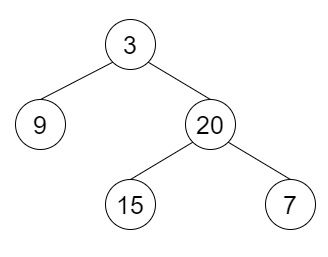# Minimum Depth of Binary Tree in C++

Suppose we have a binary tree; we have to find the minimum depth of that tree. As we know the minimum depth is the number of nodes along the shortest path from the root node down to the nearest leaf node.

So, if the input is likethen the output will be 2

To solve this, we will follow these steps −

• Define an array aa of tree nodes

• insert root at the end of aa

• Define another array ak of tree nodes

• level := 0

• if root is null, then −

• return 0

• while size of aa is not equal to 0, do −

• clear the array ak

• (increase level by 1)

• for all node a in aa −

• if (left of a is null) and (right of a is null), then −

• return level

• Come out from the loop

• if left of a is not null, then −

• insert left of a at the end of ak

• if right of a is not null, then −

• insert right of a at the end of ak

• aa := ak

• return 0

## Example

Let us see the following implementation to get a better understanding −

Live Demo

#include <bits/stdc++.h>
using namespace std;
class TreeNode{
public:
int val;
TreeNode *left, *right;
TreeNode(int data){
val = data;
left = NULL;
right = NULL;
}
};
void insert(TreeNode **root, int val){
queue<TreeNode*> q;
q.push(*root);
while(q.size()){
TreeNode *temp = q.front();
q.pop();
if(!temp->left){
if(val != NULL)
temp->left = new TreeNode(val);
else
temp->left = new TreeNode(0);
return;
}
else{
q.push(temp->left);
}
if(!temp->right){
if(val != NULL)
temp->right = new TreeNode(val);
else
temp->right = new TreeNode(0);
return;
}
else{
q.push(temp->right);
}
}
}
TreeNode *make_tree(vector<int> v){
TreeNode *root = new TreeNode(v);
for(int i = 1; i<v.size(); i++){
insert(&root, v[i]);
}
return root;
}
class Solution {
public:
int minDepth(TreeNode* root) {
vector<TreeNode*> aa;
aa.push_back(root);
vector<TreeNode*> ak;
int level = 0;
if (root == NULL || root->val == 0) {
return 0;
}
while (aa.size() != 0) {
ak.clear();
level++;
for (TreeNode* a : aa) {
if ((a->left == NULL || a->left->val == 0)&& (a->right == NULL || a->right->val == 0)) {
return level;
break;
}
if (a->left != NULL) {
ak.push_back(a->left);
}
if (a->right != NULL) {
ak.push_back(a->right);
}
}
aa = ak;
}
return 0;
}
};
main(){
Solution ob;
vector<int&g; v = {3,9,20,NULL,NULL,15,7};
TreeNode *root = make_tree(v);
cout << (ob.minDepth(root));
}

## Input

{3,9,20,NULL,NULL,15,7}

## Output

2